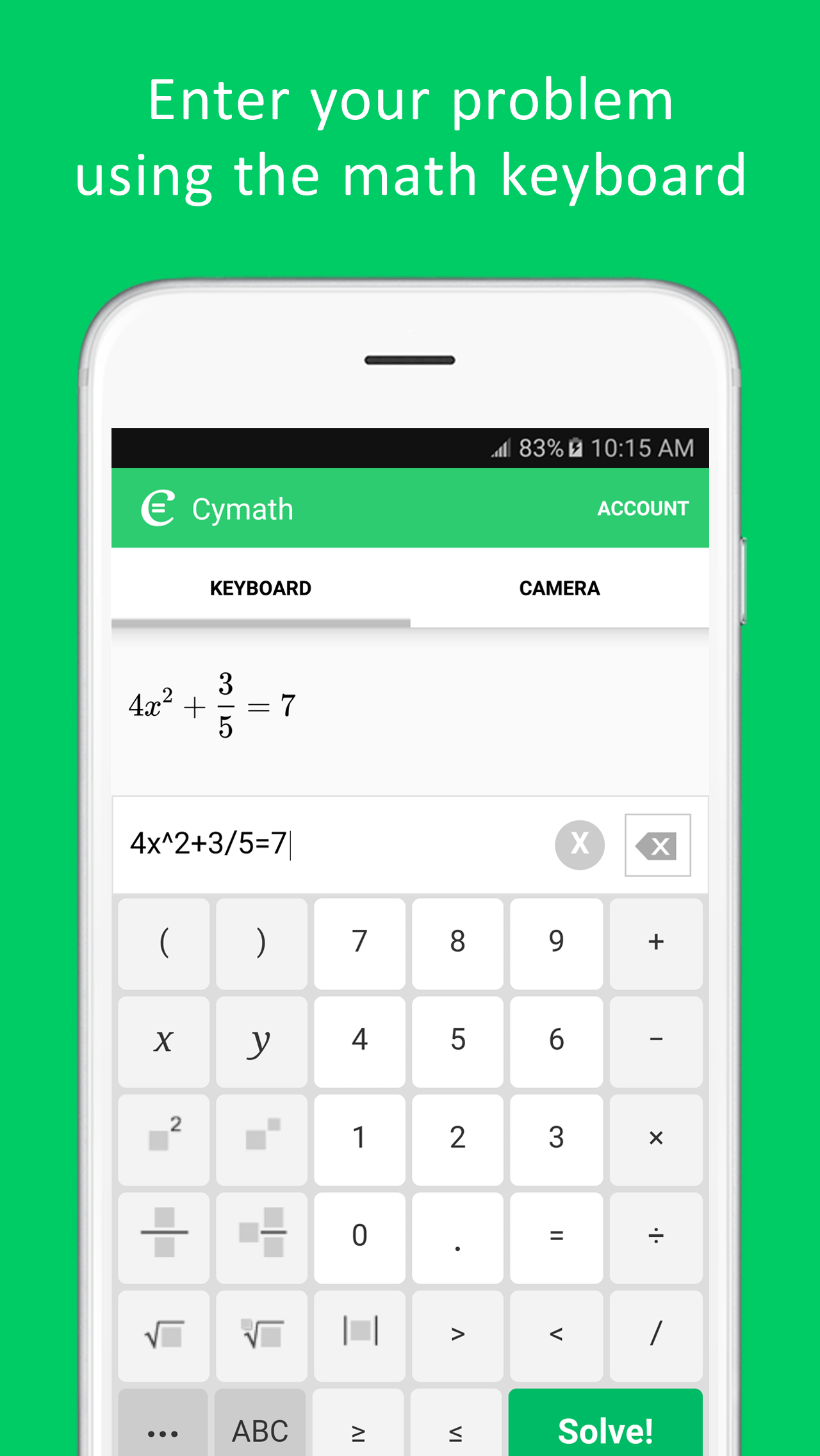# CymathName Cymath … Cymath LLC Education 2.44 2.44 1,000,000 USK: All ages December 29, 2020

Rate this post

With millions of Cymath.com users worldwide, the Cymath math problem solver app uses the same math engine while letting you solve problems on the go! Just enter a problem from your math homework, and let Cymath solve it for you step-by-step! We provide algebra as well as calculus help.

Topics in algebra include: equation solving, factoring, logarithms, exponents, complex numbers, quadratic equations, trigonometry, partial fraction, polynomial division, etc. Topics in calculus include: product rule, quotient rule, chain rule, u-substitution, integration by parts, integration by partial fraction, trigonometric substitution, rationalizing substitution, and much more. We provide math answers and steps for all of the above.

In short, whether you are simply stuck on your math homework, want to check your homework answers, or need specific calculus help, let our math problem solver help now!

## Whats New

The Cymath app now loads faster!

4.5 🧑 52,553 total
5 ⭐
97
4 ⭐
45
3 ⭐
74
2 ⭐
34
1 ⭐
30